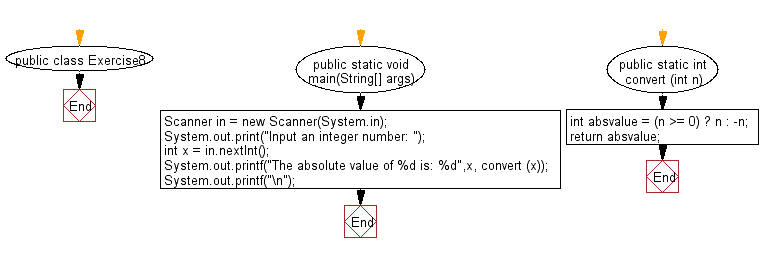﻿ Java Math Exercises: Convert an integer value to absolute value - w3resource# Java Math Exercises: Convert an integer value to absolute value

## Java Math Exercises: Exercise-8 with Solution

Write a Java program to convert an integer value to absolute value.

Sample Solution:

Java Code:

``````import java.util.*;
public class Exercise8 {
public static void main(String[] args)
{
Scanner in = new Scanner(System.in);
System.out.print("Input an integer number: ");
int x = in.nextInt();
System.out.printf("The absolute value of %d is: %d",x, convert (x));
System.out.printf("\n");
}
public static int convert (int n)
{
int absvalue = (n >= 0) ? n : -n;
return absvalue;
}
}
``````

Sample Output:

```Input an integer number: 25
The absolute value of 25 is: 25
```

Flowchart:Java Code Editor:

Improve this sample solution and post your code through Disqus

What is the difficulty level of this exercise?

﻿

## Java: Tips of the Day

Parsing dates:

```import java.io.*;
import java.util.*;
import java.text.*;

String s = "2001/09/23 14:39";

SimpleDateFormat formatter = new SimpleDateFormat ("yyyy/MM/dd H:mm");
Date d = formatter.parse(s, new ParsePosition(0));
```